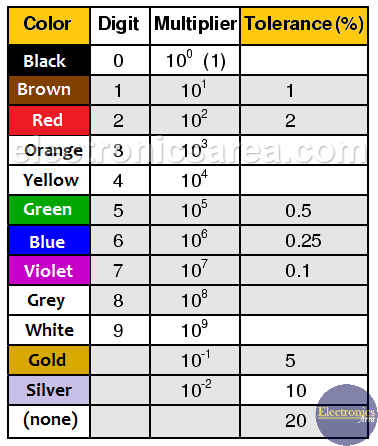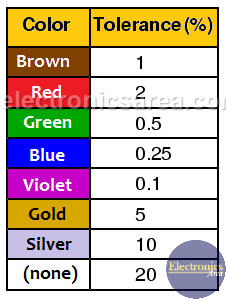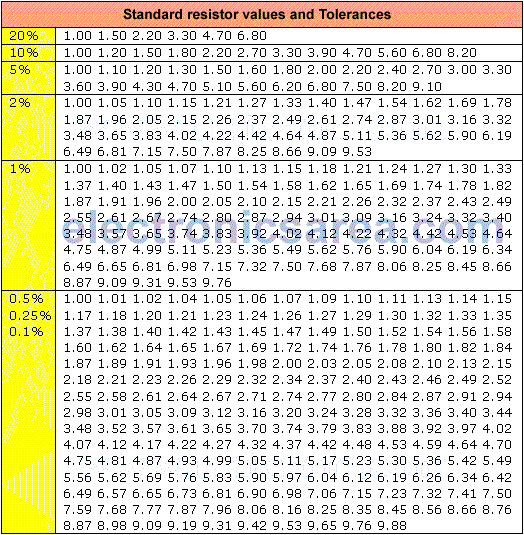# What is a resistor tolerance?

The resistor tolerance is data that tells us the percentage that can vary the resistance value of its proposed value. This variation may go up or down in the percentage specified tolerance. Typical tolerance values are 5%, 10% and 20%, but also 0.1%, 0.25%, 0.5%, 1%, 2%, 3% and 4%.

The representation of the resistor tolerance can be seen on the resistor color code. The fourth band of the code indicates the value of tolerance.Standard resistor color codeResistance tolerance color code

For example:

• If bands colors are: brown, red, yellow and gold, then resistance is: 120 Kilo Ohms +/- 5%. This resistor may have a value between 114 and 126 ohms.
• If bands colors are: brown, black, red and silver,  then resistance is: 1 Kilo Ohms +/- 10%. This resistor may have a value between 900 and 1100 ohms (1.1 Kilo Ohms).If a resistor does not have the fourth band, it is assumed that tolerance is 20%. For Example:

• If bands colors are: brown, black and red with no fourth band. Resistance is 1000 ohms, with a tolerance of 20%. This resistor can have a value between 800 and 1200 ohms.
• If bands colors are: red, red and yellow with no fourth band. Resistance is 220 Kilo Ohms, with a tolerance of 20%. This resistor can have a value between 176 Kilo Ohms and 264 Kilo Ohms.

Resistors with low tolerances are precision resistors. These resistors are used in circuits in the areas of avionics, control, measurement equipment, etc .

## What is the preferable tolerance?

High tolerance means a higher percentage of error in the resistance value and low tolerance means a low percentage of error. So low tolerance is better.

## Standard resistor values and tolerances

Standard resistor values are: 1.0, 1.2, 1.5, 1.8, 2.2, 2.7, 3.3, 3.9, 4.7, 5.6, 6.8, 8.2, etc, all x 10n, where n = 0, 1, 2, 3, 4, 5, 6.

Below there is a table with standard resistor values. These values are shown for different cases of tolerance.•
•
• 3
•
•
•
•
•
3
Shares
•
3
Shares
•
•
• 3
•
•
•
•